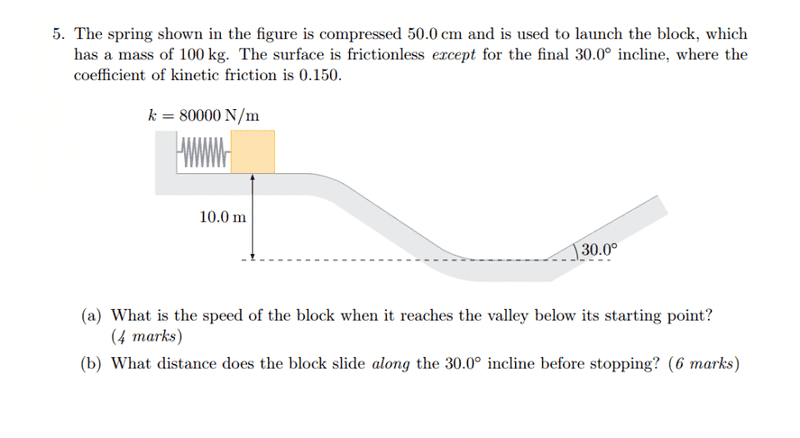# What distance does the block slide?

JiJiasd

## Homework Statement## Homework Equations

m*v^2 / 2 and k*x^2 / 2 and 0,5*m*v^2

## The Attempt at a Solution

[/B]
in task a I got correct answer there v = 20 m/s
in task b I got wrong answer I got the distance = 0,76 m but I should be 32 m

0,5*m*(sin(30)*v) - mu * m*g*cos(30) = m*g*h
h=0,38 m
sin (30) = h/L there L is the distance of the block when it goes up
L= h/sin(30) = 0,76

What I do wrong in task b ?

Thanks

Last edited by a moderator:

Replusz
0,5*m*(sin(30)*v)
sin(30)v should be squared

sidt36
I seem to get the answer on the second one to be 35.4012435624

Replusz
mu * m*g*cos(30)
Shouldnt this be mu*(m*g*cos(30))*h/cos(30)=mu*m*g*h?
I think so...

JiJiasd
I seem to get the answer on the second one to be 35.4012435624
What equation you did use ? thanks

JiJiasd
sin(30)v should be squared
I tried with 0,5*m*(sin(30)*v)^2 - mu * m*g*cos(30) = m*g*h
but it does not give me the correct answer
thanks

JiJiasd
Shouldnt this be mu*(m*g*cos(30))*h/cos(30)=mu*m*g*h?
I think so...
Now I tried 0,5*m*(sin(30)*v)^2 - mu * m*g*cos(30) *h/sin(30) = m*g*h
but not working

sidt36
I used a kinematics equation